# Complex number coordinates

Which coordinates show the location of -2+3i

x =  -2
y =  3

### Step-by-step explanation: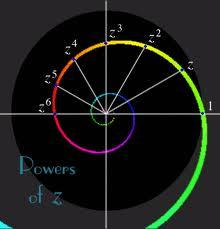Did you find an error or inaccuracy? Feel free to write us. Thank you!

Showing 1 comment:Math student
z=6*2iTips to related online calculators

## Related math problems and questions:

• Linear imaginary equationGiven that 2(z+i)=i(z+i) "this is z star" Find the value of the complex number z.
• Mappings of complex numbers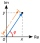Find the images of the following points under mappings: z=3-2j w=2zj+j-1
• Distance two imaginary numbsFind the distance between two complex number: z1=(-8+i) and z2=(-1+i).
• ReciprocalCalculate reciprocal of z=0.8-1.8i:
• Modulus and argumentFind the mod z and argument z if z=i
• Is complexAre these numbers 2i, 4i, 2i + 1, 8i, 2i + 3, 4 + 7i, 8i, 8i + 4, 5i, 6i, 3i complex?
• Log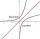Calculate value of expression log |3 +7i +5i2| .
• The modulus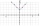Find the modulus of the complex number 2 + 5i
• Center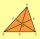In the triangle ABC is point D[1,-2,6], which is the center of the |BC|, and point G[8,1,-3], which is the center of gravity of the triangle. Find the coordinates of the vertex A[x,y,z].
• De Moivre's formula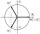There are two distinct complex numbers z, such that z3 is equal to 1 and z is not equal to 1. Calculate the sum of these two numbers.
• Linear combination of complex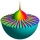If z1=5+3i and z2=4-2i, write the following in the form a+bi a) 4z1+6z2 b) z1*z2
• ABS CNCalculate the absolute value of complex number -15-29i.
• LineStraight-line passing through points A [-3; 22] and B [33; -2]. Determine the total number of points of the line in which both coordinates are positive integers.
• Evaluate 18Evaluate the expression (-4-7i)-(-6-9i) and write the result in the form a+bi (Real + i* Imaginary).
• Goniometric form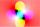Determine the goniometric form of a complex number z = √ 110 +4 i.
• Coordinates of a centroindLet’s A = [3, 2, 0], B = [1, -2, 4] and C = [1, 1, 1] be 3 points in space. Calculate the coordinates of the centroid of △ABC (the intersection of the medians).
• CoordinatesDetermine the coordinates of the vertices and the content of the parallelogram, the two sides of which lie on the lines 8x + 3y + 1 = 0, 2x + y-1 = 0 and the diagonal on the line 3x + 2y + 3 = 0